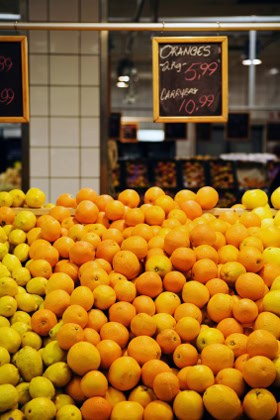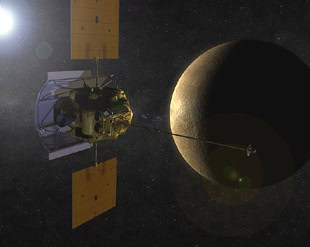What does stacking shelves in a supermarket have in common with beaming messages from space probes? Unlikely as it sounds, both are governed by the same mathematical problem: sphere-packing. No matter how tightly you push a collection of spheres together there will always be some leftover space in between, and figuring out exactly how much has kept mathematicians busy for years.

### Circles and hexagons

Next time you’re browsing the aisles at your local supermarket, take a look at the tinned goods section. The tins on the shelves are probably lined up in neat rows and columns, an arrangement that mathematicians call a square lattice. Storing tins like this keeps them nice and ordered, but it isn’t actually the best use of the shelf space available. The most efficient way to pack circles, such as the base of a tin, is a hexagonal lattice: each tin surrounded by exactly six others. But how do we know that’s the most efficient arrangement?

It’s easy to show why hexagons beat squares square. Circles only coveror around 78%, of a square lattice, but they coverof a hexagonal lattice, more than 90%. In the early 19th century the German mathematician Carl Friedrich Gauss proved that no other regular lattice could do any better, but he didn’t completely solve the problem. What if there was some random arrangement of circles out there that was more efficient than hexagons? It wasn’t until 1940 that the Hungarian mathematician László Fejes-Tóth settled it once and for all, proving that hexagons are always best.

### Sphere StackingOf course, mathematicians are never content with just two dimensions. Walk across to your supermarket’s fresh fruit section and you’ll find stacks of orange, melons, and other round fruits – sphere-packing in three dimensions. The most intuitive way of arranging spheres starts with a hexagonal lattice then stack another above it, with the layer on top nestling in the gaps of the layer below. Each sphere ends up touching 12 others; six horizontally, and three above and below. This arrangement is called the face-centered cubic lattice, and fillsor about 74% of the total volume. In comparison, stacking the spheres in a regular grid known as a cubic lattice only takes up, or about 52%.

As with circles, mathematicians wondered if they could do any better, but in 1611 a German mathematician and astronomer named Johannes Kepler suggested they couldn’t. He wrote a paper investigating how different arrangements of spheres and circles could explain patterns in the natural world such as the six-fold symmetry of a snowflake. His claim that the face-centered cubic lattice is the most efficient packing became known as the Kepler conjecture, a puzzle that remained unsolved for nearly 400 years.

It wasn’t for lack of trying. Along with demonstrating the superiority of the hexagonal lattice in two dimensions, Gauss also proved that no regular arrangement of spheres could beat the face-centered cubic lattice. In the 1950s, Fejes-Tóth showed that you don’t need an infinite number of spheres to check all the irregular patterns, but just 50. Finally in 1998, an American mathematician named Thomas Hales claimed to have proved Kepler right. The proof involved checking the packing efficiency of over 5,000 different arrangements of spheres and required more than 100,000 computer calculations. Like other computer-aided proofs, some mathematicians are a little wary of confirming Kepler’s conjecture just yet, but most accept it as true.

### Out of this worldBy now you’re probably wondering what any of this has to do space probes, but you won’t find the answer in two or three dimensions. Surprisingly, it’s 24-dimensional spheres that hold the key to communicating across the vastness of space. Though we can’t easily visualise them, the mathematical description of a 24-dimensional sphere is exactly the same as a three-dimensional one, but with more numbers. The equation for a sphere of radius r is x2 + y2 + z2 = r2, and just as the coordinates (x, y, z) denote a point in 3D space, a list of n numbers corresponds to a point in n-dimensional space.

Space probe computers also use lists of numbers. Like all computers, they store data as a string of 0’s and 1’s called binary, and to make sense of these lists you have to choose a particular binary code. For example, the standard code for the letter A is 01000001. We can also choose to interpret this code in another way, as coordinates in 8-dimensional space, and that’s where sphere-packing comes in.

Spheres in 24 dimensions pack extremely well in a highly symmetrical arrangement called the Leech lattice. Each sphere touches exactly 196,560 others in a pattern that turns out to be useful in generating error-correcting binary codes. Using the coordinates at the centre of each 24-dimensional sphere as a binary code means that there is no overlap between them, and it doesn’t matter if errors creep into a string of 24 binary digits as it’s transmitted. If a few 0s get turned into 1s, the result will still be inside the right sphere, and we can correct the error to find the original binary string.

NASA used this method of communication, called a Golay code, to beam pictures of Jupiter and Saturn from the Voyager space probes in the late 1970s, and similar techniques power all kinds of digital transmissions today. Kepler would be proud.